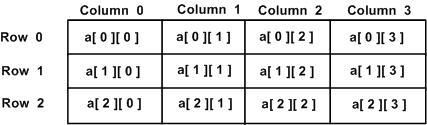# Multidimensional Arrays

In the previous tutorials on Arrays, we covered, well, arrays and how they work. The arrays we looked at were all one-dimensional, but C can create and use multi-dimensional arrays. Here is the general form of a multidimensional array declaration:

``````type name[size1][size2]...[sizeN];
``````

For example, here's a basic one for you to look at -

``````int foo;
``````

or maybe this one -

``````char vowels = {
{'a', 'e', 'i', 'o', 'u'}
};
``````

### Two-dimensional Arrays

The simplest form of multidimensional array is the two-dimensional array. A two-dimensional array is pretty much a list of one-dimensional arrays. To declare a two-dimensional integer array of size [ x ][ y ], you would write something like this −

``````type arrayName [x][y];
``````

Where type can be any C data type (int, char, long, long long, double, etc.) and arrayName will be a valid C identifier, or variable. A two-dimensional array can be considered as a table which will have [ x ] number of rows and [ y ] number of columns. A two-dimensional array a, which contains three rows and four columns can be shown and thought about like this −In this sense, every element in the array a is identified by an element name in the form a[i][j], where 'a' is the name of the array, and 'i' and 'j' are the indexes that uniquely identify, or show, each element in 'a'.

And honestly, you really don't have to put in a [ x ] value really, because if you did something like this -

``````char vowels[] = {
{'A', 'E', 'I', 'O', 'U'},
{'a', 'e', 'i', 'o', 'u'}
};
``````

the compiler would already know that there are two "dimensions" you could say, but, you NEED a [ y ] value!! The compiler may be smart, but it will not know how many integers, characters, floats, whatever you're using you have in the dimensions. Keep that in mind.

### Initializing Two-Dimensional Arrays

Multidimensional arrays may be used by specifying bracketed[] values for each row. Below is an array with 3 rows and each row has 4 columns. To make it easier, you can forget the 3 and keep it blank, it'll still work.

``````int a = {
{0, 1, 2, 3} ,   /*  initializers for row indexed by 0 */
{4, 5, 6, 7} ,   /*  initializers for row indexed by 1 */
{8, 9, 10, 11}   /*  initializers for row indexed by 2 */
};
``````

The inside braces, which indicates the wanted row, are optional. The following initialization is the same to the previous example −

``````int a = {0,1,2,3,4,5,6,7,8,9,10,11};
``````

### Accessing Two-Dimensional Array Elements

An element in a two-dimensional array is accessed by using the subscripts, i.e., row index and column index of the array. For example −

``````int val = a;
``````

The above statement will take the 4th element from the 3rd row of the array.

## Exercise

Let us try to find out the average marks of a group of five students for two subjects, Mathematics and Physics. To do this, we use a two-dimensional array called `grades`. The marks corresponding to Mathematics would be stored in the first row (`grades`), whereas those corresponding to Physics would be stored in the second row (`grades`). Complete the following steps so that you can execute this program.

• Declare grades as a two-dimensional array of integers
• Complete the for loops by specifying their terminating conditions
• Compute the average marks obtained in each subject